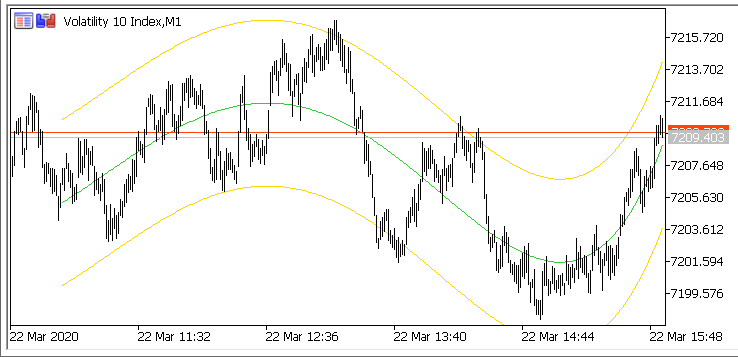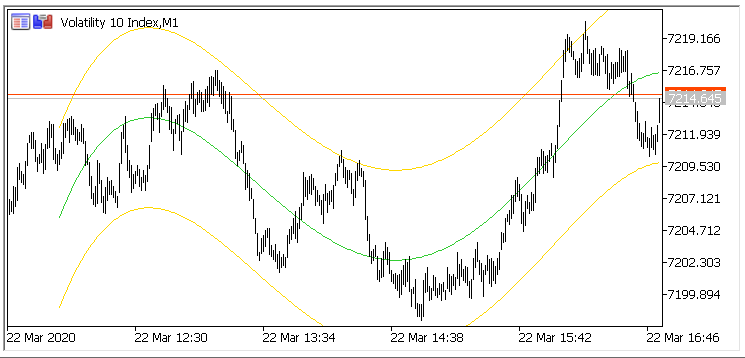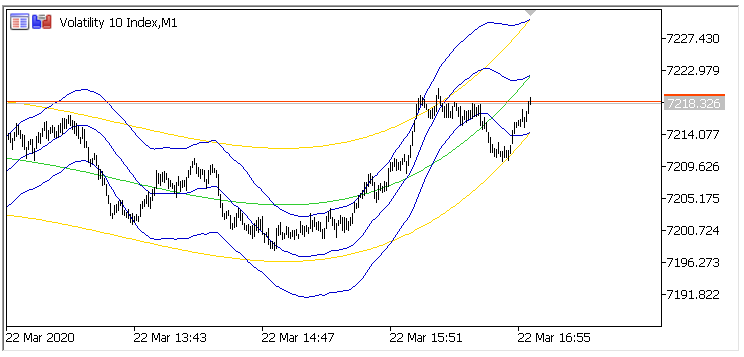# Regression Channel with variable polynomial degree – indicator for MetaTrader 5

A quick port for MT5.

Real authors:
original by Boris: https://www.mql5.com/en/code/8417
V1.1
by graziani: https://www.mql5.com/en/code/11749

• i-Regr: Polynomial Regression Channel consists of two parallel lines, equidistant up and down from the line of polynomial regression
trend. The distance between frame of the channel and regression line equals to the value of maximum close price deviation from the
regression line.
If price lower than under line – Buy, If price bigger than upper line – Sell, TakeProfit by average line.Screenshot an hour later:The indicator is a great tool to talk about statistics of historical data.

The right edge history (blue lines) is not that convincing:## Regression Channel with variable polynomial degree, Indicator & EA – indicator for MetaTrader 4

Real author: Boris, www.iticsoftware.com This is a fast adaptation of old source to MetaTrader 4 b600 environment. i-Regr: Linear Regression Channel consists of two parallel…

## Regression Сhannel – indicator for MetaTrader 4

The channel indicator plots a linear regression channel. First the indicator draws a trend line between two dots, then on its basis plots a channel…

## Regression Сhannel – indicator for MetaTrader 4

The channel indicator plots a linear regression channel. First the indicator draws a trend line between two dots, then on its basis plots a channel…

## Simple Regression Channel – indicator for MetaTrader 4

Simple Regression Channel automatically generates Regression Channel, based on timeframe (user input). Linear Regression Channel consists of two parallel lines located at the equal distance…

## i-Regr – indicator for MetaTrader 5

Platform: Metatrader5 Currency pairs: Any pairs, indices and metals Trading Time: Around the clock Recommended broker: icmarkets, XM Regression Channel The Linear Regression Channel consists of…